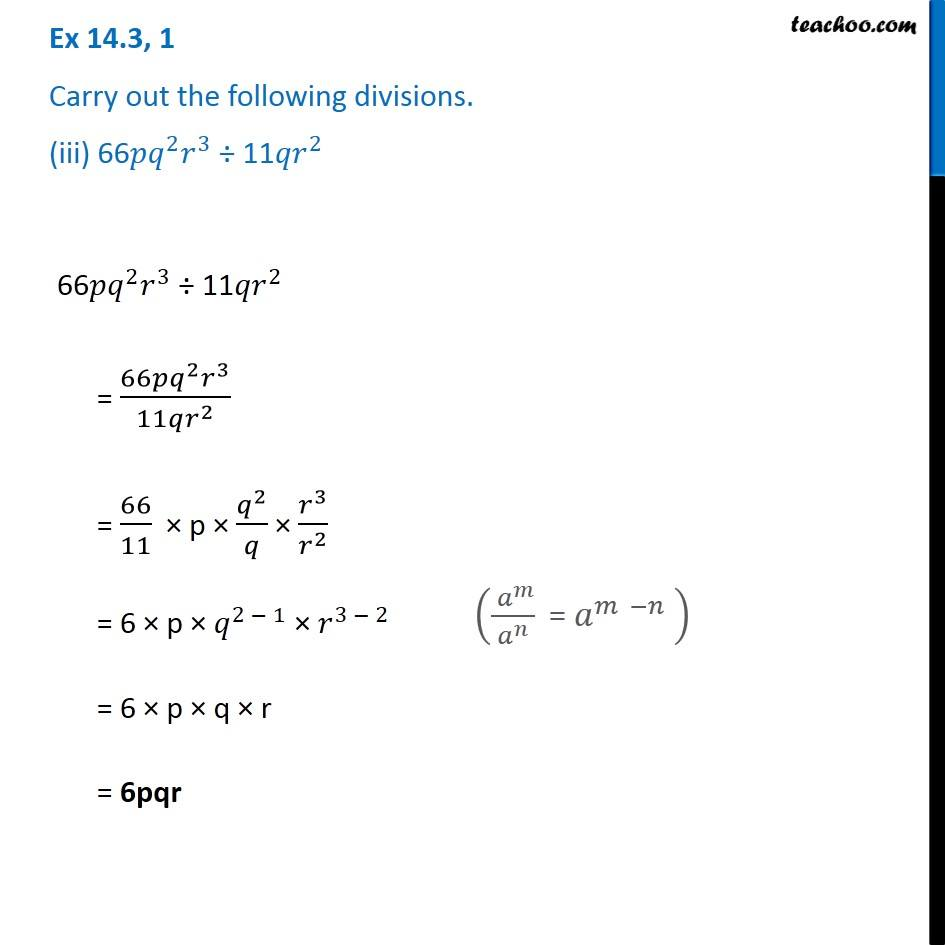1. Chapter 14 Class 8 Factorisation
2. Concept wise
3. Dividing two monomials

Transcript

Ex 14.3, 1 Carry out the following divisions. (iii) 66〖𝑝𝑞〗^2 𝑟^3 ÷ 11〖𝑞𝑟〗^2 66〖𝑝𝑞〗^2 𝑟^3 ÷ 11〖𝑞𝑟〗^2 = (66𝑝𝑞^2 𝑟^3)/(11𝑞𝑟^2 ) = 66/11 × p × 𝑞^2/𝑞 × 𝑟^3/𝑟^2 = 6 × p × 𝑞^(2 − 1) × 𝑟^(3 − 2) = 6 × p × q × r = 6pqr (( 𝑎^𝑚)/𝑎^𝑛 " = " 𝑎^(𝑚 −𝑛) " " )